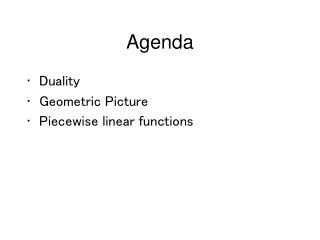DownloadDownload PresentationAgenda

# Agenda

Télécharger la présentation## Agenda

- - - - - - - - - - - - - - - - - - - - - - - - - - - E N D - - - - - - - - - - - - - - - - - - - - - - - - - - -
##### Presentation Transcript

1. Agenda • Duality • Geometric Picture • Piecewise linear functions

2. Dual Problem Original: max profit from running plant s.t. capacity not exceeded variables are production quantities Dual: min cost to buy all capacity s.t. willing to sell capacity instead of produce variables are prices

3. Dual Problem Original: max \$840 profit * S cars + … s.t. 3hr * S + 2hr * F + 1hr * L <= 120hr engine shop capacity 1hr * S + 2hr * F + 3hr * L <= 80hr body shop capacity … variables S, F, L are production quantities Dual: min price E * 120 hr engine shop capacity + … s.t. 3hr * E + 1hr * B + 2hr * SF >= \$840 (standard car profit) 2hr * E + 2hr * B + 3hr * FF >= \$1120 (fancy car profit) … variables E, B, SF, FF, FL are prices

4. Results • constraint becomes dual variable • constraint bound goes into dual objective • shadow price = optimal dual variable • variable becomes dual constraint • objective coefficient is dual constraint bound • optimal value = dual shadow price • max problem becomes min problem • solutions the same • unbounded problem becomes infeasible

5. Generic Dual Problem maxx pTx s.t. Ax <= c x >= 0 equivalent to miny cTy s.t. ATy >= p y >= 0

6. Electric Utility Example • Customer demand d • Generator i has cost ci and capacity bi • Production xi on generator i • Goal: meet demand with little cost minx cTx s.t. x1+x2+…+xn >= d xi <= bi for i=1,..,n x >= 0

7. Electric Utility Example Original: minx cTx s.t. x1+x2+…+xn >= d xi <= bi for i=1,…,n x >= 0 Dual: maxp,y dp - bTy s.t. p - yi <= ci for i=1,…,n p >= 0, y >= 0

8. Electric Utility Example Dual maxp,y dp - bTy s.t. p - yi <= ci for i=1,…,n p >= 0, y >= 0 p = market price for power yi = profit rate at generator i constraint: yi >= p - ci Goal: max net revenue (after paying out-sourced generators their profit)

9. Manipulations • min f(x) = - max -f(x) • g(x) <= b same as -g(x) >= -b • x <= 5 same as -x >= -5

10. General Dual Formulation maxx pTx s.t. Ax ? c x ? 0 miny cTy s.t. ATy ? p y ? 0 • for max problem <= constraint becomes variable >= 0 >= constraint becomes variable <= 0 = constraint becomes variable without bound • for min problem the opposite

11. Piecewise Linear Functions minx c1(x1) + c2x2 s.t. x1+x2 >= d x >= 0 minx,z z + c2x2 s.t. x1+x2 >= d x >= 0 z >= s1 x1 z >= s2 x1 + t c1(x1)